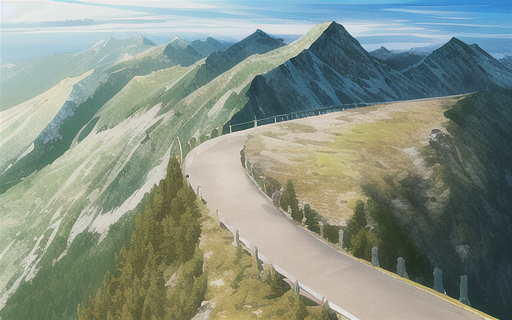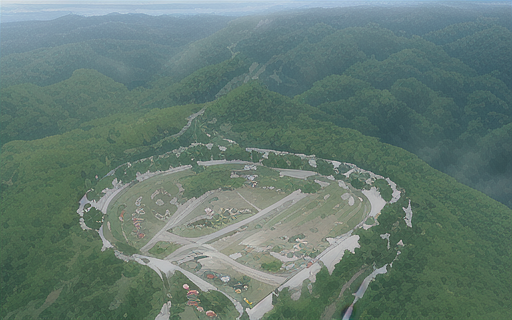# Will the box tip over?

2023-10-05 #zmp #kkt #dynamics

Think of a box with a mass $$m$$ on the ground, with some force $$f$$ and a torque $$\tau$$ applied to it (it can be the gravity, but also some external forces applied on it like somebody pushing it).

What happens to the box? Your brain effortlessly gives you some possible answers to that question: it might stay in place, it might tip, it might slide, it might be lifted up… How do we determine what actually happens?

Suppose first that the box is free (no obstacle or ground). If its mass is $$m$$ and its inertia $$I$$, and it is subject to forces $$f_x$$, $$f_y$$ and $$\tau$$ (torque) the equation of motion can then directly be deduced from Newton’s second law:

$\underbrace{ \begin{bmatrix} f_x \\ f_y \\ \tau \end{bmatrix} }_{F} = \underbrace{ \begin{bmatrix} m & 0 & 0 \\ 0 & m & 0 \\ 0 & 0 & I \end{bmatrix} }_{M} \underbrace{ \begin{bmatrix} \ddot x \\ \ddot y \\ \ddot \theta \end{bmatrix} }_{\ddot X}$

In this case, the acceleration is then $$\ddot X^{free} = M^{-1} F$$.

# Gauss’ principle of least constraint

The presence of the ground introduces a constraint on our motion, in particular, the box can’t penetrate the ground, which will intuitively prevent the box to accelerate downwards.

As you can guess, this constraint will eventually result in extra forces that will end up in the equation of motion, affecting our acceleration. But how do we compute those forces? And how can wether the box will tilt or not?

We can here use a powerful tool: Gauss’ principle of least constraint. According to this principle, the true acceleration will be the one minimizing the following quantity:

$\begin{equation} \begin{split} \min_{\ddot X} \space (\ddot X - \ddot X^{free})^T M (\ddot X - \ddot X^{free}) \\ \text{with} \space \ddot X \space \text{subject to constraints} \end{split} \end{equation}$

# Unconstrained optimization

First things first, we can check what happens with this optimization problem if there is no constraint. Substituting $$\ddot X^{free}$$ with $$M^{-1} F$$ in the objective function mentioned previously, the value to minimize becomes:

$\begin{equation} \begin{split} & (\ddot X - M^{-1} F)^T M (\ddot X - M^{-1} F) \\ & = (\ddot X^T - F^T M^{-T}) M (\ddot X - M^{-1} F) \\ & = (\ddot X^T M - F^T) (\ddot X - M^{-1} F) \\ & = \ddot X^T M \ddot X - 2 F^T \ddot X + F^T M^{-1} F \end{split} \end{equation}$

Since our optimization variable is $$\ddot X$$, the last term ($$F^T M^{-1} F$$) is constant and can be ignored. The objective can then be rewritten as:

$\frac{1}{2} \ddot X^T M \ddot X - F^T \ddot X$
Mountain metaphorHow do we minimize such an equation? We will for that use a common metaphor: imagine that you are on a mountain, and want to find the lowest point. We are for now unconstrained and can go anywhere we want.

The mountain can be vast, and you can't explore the whole of it, so there is no way to find this point for sure, you can however define a necessary condition for a point to be the lowest: the slope of the mountain has to be locally flat at this point (else, there would be a direction to get even lower).

In other words, the gradient of the objective has to be zero. In our case:

$\frac{\partial}{\partial \ddot X} \left( \frac{1}{2} \ddot X^T M \ddot X - F^T \ddot X \right) = 0$

That we can compute as:

$M \ddot X - F = 0$

Which will yeld $$\ddot X = \ddot X^{free} = M^{-1} F$$ as expected. (Since our objective is quadratic, it only has one stationary point, which is the minimum.)

# Equality constrained optimization

Before considering the ground constraint, let’s start with a simpler one: suppose the box can’t move up and down. Imagine it being on some tracks, it can translate along the $$x$$ axis or rotate, but not move up and down along the $$y$$ axis.

The constraint is then $$\ddot y = 0$$, which we can write in matrix form as:

$\underbrace{ \begin{bmatrix} 0 & 1 & 0 \end{bmatrix} }_A \ddot X = \underbrace{0}_b$
Mountain metaphorThis is an equality constraint. If you go back to the mountain metaphor, you can think of it as the fact that you have to stay on a road. You now need to find the lowest point on the mountain that can be accessed on that road.

The previous condition needs to be adapted, the slope of the mountain doesn't have to be flat anymore, it is possible that the road simply prevents us from getting any lower, because it is orthogonal to its slope.

In mathematical terms, this means that the gradient of the objective function has to be collinear to the gradient of the constraint. This means that there is a scalar $$\lambda$$ such that:

$M \ddot X - F + \lambda A^T = 0$

This scalar is a new unknown called a Lagrange multiplier. Packaging everything together in a matrix, we can now rewrite our problem as:

$\begin{bmatrix} M & A^T \\ A & 0 \end{bmatrix} \begin{bmatrix} \ddot X \\ \lambda \end{bmatrix} = \begin{bmatrix} F \\ b \end{bmatrix}$

The matrix on the left is sometime referred to as the KKT matrix (for Karush-Kuhn-Tucker). If we go back to our example where $$\ddot y = 0$$, the equation becomes:

$\begin{bmatrix} m & 0 & 0 \\ 0 & m & 0 \\ 0 & 0 & I \end{bmatrix} \begin{bmatrix} \ddot x \\ 0 \\ \ddot \theta \end{bmatrix} = \begin{bmatrix} f_x \\ f_y \\ \tau \end{bmatrix} + \begin{bmatrix} 0 \\ \lambda \\ 0 \end{bmatrix}$

In this equation, $$\lambda$$ has the unit of a force, and has to counter $$f_y$$ to cancel it out and enforce the constraint. The beauty of this is: we start with geometrical constraints, and end up with constraint forces.

# Inequality constrained optimizationBack to the original problem: will the box tip over? We can now answer this question by defining the appropriate constraints:

• The box can’t slide,
• The box can’t penetrate the ground.

If we call $$A$$ and $$B$$ the left and right corners of the box, the non-sliding constraint can be defined as $$\ddot x_A = \ddot x_B = 0$$.

However, the non-penetration constraint is trickier, since $$\ddot y_A = \ddot y_B = 0$$ would prevent the box from being lifted up. What we need here is $$\ddot y_A \geq 0$$ and $$\ddot y_B \geq 0$$, which are inequality constraints. (Note that ensuring those constraints ensure that all the contact points on the $$[A,B]$$ segment also have an upward acceleration.)

Mountain metaphorBack again to the mountain metaphor, now think of the road as a parking lot, you can drive anywhere in it, but can't cross its borders.

The lowest point of the mountain could be somewhere in this parking, which means a flat slope as in the unconstrained problem, but it could also be on the border of the parking. This second case is kind of similar with the road, with the difference that we need the slope to go lower outwards the parking (because, else, we could go lower by going inwards which we are allowed to).

In mathematical terms, you then have two cases for each inequality constraint:

1. Either it is not limiting the optimal solution. To assess that, you could solve the problem without taking this constraint in account, and check that the optimal solution satisfies it.
2. Or it is indeed limiting the optimal solution , you can then treat it as an equality constraint, and check that the sign of the associated Lagrange multiplier is positive for a $$\leq$$ constraint, or negative for a $$\geq$$ constraint.

Solving such a problem requires finding which inequalities are in case 1 or 2. For quadratic objective functions and linear constraints, this work is efficiently done by quadratic programming solver.

Having two inequalities for the box problem, we have four possible such combinations, that all correspond to an intuitive physical outcome:

1. $$\ddot y_A > 0$$ and $$\ddot y_B > 0$$: the box is lifted up,
2. $$\ddot y_A > 0$$ and $$\ddot y_B = 0$$: the box is tilting to the right,
3. $$\ddot y_A = 0$$ and $$\ddot y_B > 0$$: the box is tilting to the left,
4. $$\ddot y_A = 0$$ and $$\ddot y_B = 0$$: the box doesn’t move.

If we want to check if the box will move, we could then enable the two constraints as equality constraints, (like in case 4.) and ensure that the Lagrange multipliers are negative.

# Putting it all together

Suppose that the box has a width of $$2l$$ and its center of mass is at height of $$h$$, the acceleration of $$A$$ and $$B$$ are:

$\begin{equation} \begin{split} \ddot x_A & = \ddot x + \ddot \theta h \\ \ddot y_A & = \ddot y - \ddot \theta l \\ \ddot x_B & = \ddot x + \ddot \theta h \\ \ddot y_B & = \ddot y + \ddot \theta l \end{split} \end{equation}$

Because $$\ddot x_A = \ddot x_B$$, the non-sliding constraint is $$\ddot x + \ddot \theta h = 0$$. Our constraint matrix $$A$$ now becomes:

$\underbrace{ \begin{bmatrix} 1 & 0 & h \\ 0 & 1 & -l \\ 0 & 1 & l \end{bmatrix} }_A \underbrace{ \begin{bmatrix} \ddot x \\ \ddot y \\ \ddot \theta \end{bmatrix}}_{\ddot X} = 0$

This obviously implies zero acceleration. The stationary condition is then:

$A^T \lambda = F$

Or in other words:

$\begin{bmatrix} 1 & 0 & 0 \\ 0 & 1 & 1 \\ h & -l & l \end{bmatrix} \begin{bmatrix} \lambda_1 \\ \lambda_2 \\ \lambda_3 \end{bmatrix} = \begin{bmatrix} f_x \\ f_y \\ \tau \end{bmatrix}$

Solving this system yields:

$\lambda_1 = f_x \\ \lambda_2 = \frac{1}{2} [ f_y - \frac{\tau}{l} + h \frac{f_x}{l} ] \\ \lambda_3 = \frac{1}{2} [ f_y + \frac{\tau}{l} - h \frac{f_x}{l} ]$

Now, remember that for this to be the optimal solution to our optimization problem, the sign of the Lagrange multipliers has to be negative (because we initially had $$\ddot y_A \geq 0$$ and $$\ddot y_B \geq 0$$ constraints), which means, that:

$\begin{equation} \begin{split} f_y + \frac{1}{l} [ \tau - h f_x ] \leq 0 \\ f_y - \frac{1}{l} [ \tau - h f_x ] \leq 0 \\ \end{split} \end{equation}$

By summing those two equations, we can deduce that $$f_y \leq 0$$. We can in that case divide by $$f_y$$ and rewrite this with the form:

$-l \leq \frac{1}{f_y} [ h f_x - \tau ] \leq l$

Here, the quantity:

$z = \frac{1}{f_y} [ h f_x - \tau ]$

Is a value that has to be in the range $$[-l, l]$$ for the box not to tip over.

Since it has the unit of meters, we can wonder if it has a physical meaning. It turns out that it does: this is the famous zero-tilting moment point (ZMP) that is used in robotics to assess the stability of a robot.

The quantity $$\tau - h f_x$$ can be thought as the moments expressed at the origin of the frame (which is here on the ground below the center of mass). The moment of any point on the ground with $$x$$ coordinate would be $$tau - h f_x + x f_y$$, which can be canceled at $$x = z$$, hence the name of the ZMP.

For the box not to tip over, this point $$z$$ has to lie inside the box:In 3D formulation, the ZMP has to lie inside the convex hull of the contact points with the ground.

# To go further

The conditions for optimality mentioned above are an intuitive glance at KKT conditions, which are a fundamental result in optimization theory.

The ZMP is an important concept in robotics to assess the stability of a robot. You can check at Stéphane Caron’s blog for further derivations.

Thanks to Stéphane Caron for proof-reading this post!